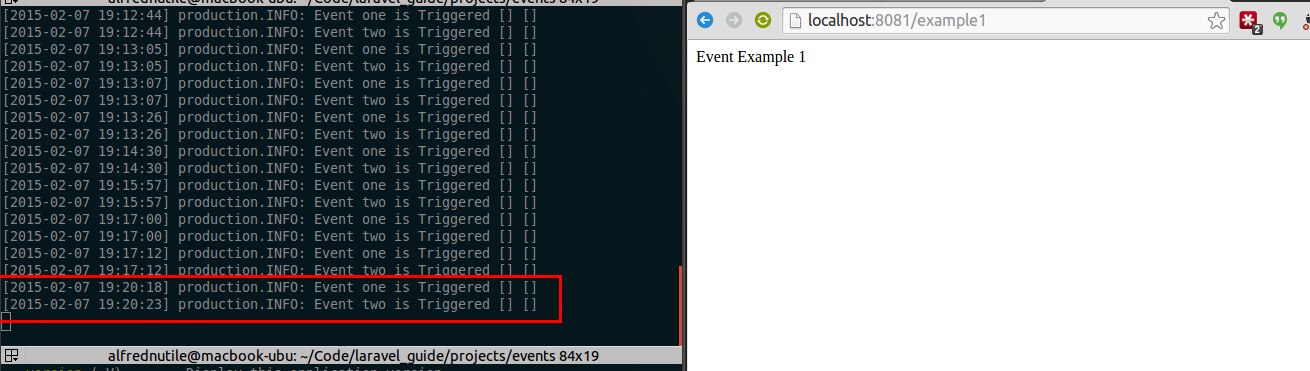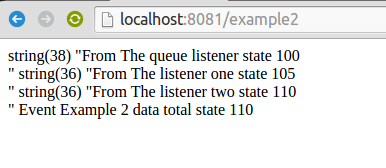# Events

## Getting Started

This is part of a larger Laravel Guide I am working on in this case the example is in the Events folder

Start the example

``````php artisan serve --port=8081
``````

Then visit

``````http://localhost:8081/example1
``````

So if you look in

``````app/routes.php
``````

You see route example1 will fire and event and there are two listeners

## Do they run async or sync

If you hit that route you see the sleep taking effect so it is syncronous

See in the image below an example. I reload the page and the results are staggered by the sleep amount in the route.On the left I have

``````php artisan tail
``````

Here is the route

``````Event::listen('example1', function()
{
sleep(5);
Log::info("Event one is Triggered");
});

Event::listen('example1', function()
{
sleep(5);
Log::info("Event two is Triggered");
});

Route::get('/example1', function()
{
Event::fire('example1');
return "Event Example 1";
});
``````

So if you did not want to hold up the process you would place these into a queue.

## Passing data to events

How can Event 1 alter Event 2 and Event 3

See route would be /example2

Also there is a registered event listener seen in the route file that

``````\$subscriber = new \Acme\ExampleEventHandler;
Event::subscribe(\$subscriber);
``````

Which shows how that made it hard to pass a state

``````\$subscriber = new \Acme\ExampleEventHandler;

Event::subscribe(\$subscriber);

Event::listen('example2', function(\$state)
{
\$state->total = \$state->total + 5;
var_dump(sprintf("From The listener one state %s <br>", \$state->total));

Log::info(sprintf("Event two listener 1 is Triggered total %s", \$state->total));
});

Event::listen('example2', function(\$state)
{
\$state->total = \$state->total + 5;
var_dump(sprintf("From The listener two state %s <br>", \$state->total));

Log::info(sprintf("Event two listener 2 is Triggered total %s", \$state->total));
});

Route::get('/example2', function()
{
\$state = new stdClass();
\$state->total = 0;
Event::fire('example2', array(\$state));

return sprintf("Event Example 2 data total state %s", \$state->total);
});
``````

This one you just see in the browser